# DAV Class 7 Maths Chapter 6 Brain Teasers Solutions

The DAV Class 7 Maths Solutions and DAV Class 7 Maths Chapter 6 Brain Teasers Solutions of Algebraic Expressions offer comprehensive answers to textbook questions.

## DAV Class 7 Maths Ch 6 Brain Teasers Solutions

Question 1.
A. Tick (S) the correct option:
(i) (3p2 – 14pq + 2r) – (14pq + 3p2 + 2r2) is a:
(a) monomial
(b) binomial
(c) trinomial
(d) none
(3p2 – 14pq + 2r) – (14pp + 3p2 + 2r2)
= 3p2 – 14pq + 2r – 14pq – 3p2 – 2r2
= -2 r2 – 28 pq + 2r
As this algebraic expression have three terms so it is a trinomial.
Hence, (c) is the correct answer.

(ii) H.C.F. of the terms of the expression (4p3q2r – 12pq2r2 + 16p2q2r2) is:
(a) 4pq2r
(b) -4pq2r
(c) 16p3q2r2
(d) -16p3q2r2
We know HCF shall be the product of the highest common coefficient and highest common powers of the variables respectively.
HCF of 4p3q2r, -12pq2r2 and 16p2q2r2 is 4pq2r.
Hence, (a) is the correct option.

(iii) For what value of’t’ the expressions (2x2 – 5x + 10) amd (2x2 – tx + 2t) are equal:
(a) 2
(b) 5
(c) 3
(d) 4
The given expressions are (2x2 – 5x + 10) and (2x2 – tx + 2t).
Now, comparing the coefficients of x, we have -5 = -t
⇒ t = 5
Also, comparing the constant terms in the given expressions, we have
10 = 21
⇒ t = $$\frac{10}{2}$$ = 5
Hence, (b) is the correct option.

(iv) Value of ‘p’ if the expression z2 + 3z – p equals to 3 for z = 2 is:
(a) 6
(b) 5
(c) 7
(d) 4
On putting the value of z = 2 in the expression z2 + 3z – p, we have
(2)2 + 3(2) – p = 3
4 + 6 – p = 3
⇒ 10 – p = 3
⇒ p = 10 – 3
⇒ p = 7
Hence, (c) is the correct option.

(v) Factors of 2x3 + 5x – 6x2 – 15 are:
(a) (2x2 + 5) and (x + 3)
(b) (2x2 – 5) and (x + 3)
(c) (2x2 + 5) and (x – 3)
(d) (2x2 – 5) and (x + 3)
= (2x3 – 6x2) + (5x – 15) = 2x2 (x – 3) + 5(x – 3)
= (x – 3)(2x2 + 5)
= (2x2 + 5) (x – 3)
Hence, (c) is the correct option.(i) In the given figure, ABCD is a rectangle with length (p2q – 5) and breadth (q2 + 4p). Find the area of shaded triangle PAB.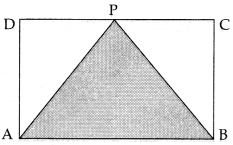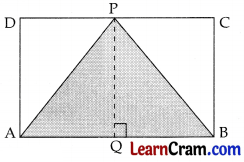Draw PQ perpendicular to AB
Here, base (AB) = (p2q – 5) and altitude (PQ) = (q2 + 4p)
∴ Area of shaded triangle PAB = $$\frac{1}{2}$$ × Base × Altitude
= $$\frac{1}{2}$$ × AB × PQ
= $$\frac{1}{2}$$ × (p2q – 5) × (q2 + 4p)
= $$\frac{1}{2}$$ (p2q – 5) (q2 + 4P)
= $$\frac{1}{2}$$[p2q[q2 + 4p) – 5(q2 + 4p)]
= $$\frac{1}{2}$$ (p2q3 + 4p3q – 5q2 – 20p) sq. units

(ii) By how much does the expression 72z2 – 45z + 4 exceed the expression 30z – 42z2 – 17?
The expression (72z2 – 45z + 4) exceeds the expression (30z – 42z2 -17) by (72z2 – 45z + 4) – (30z – 42z2 – 17).
Now, subtracting by horizontal method, we have (72z2 – 45z + 4) – (30z – 42z2 – 17)
= 72z2 – 45z + 4 – 30z + 42z2 + 17
= (72z2 + 42z2) + (-45z – 30z) + (4 + 17)
= 114z2 – 75z + 21
So, the expression (72z2 – 45z + 4) exceeds the expression (30z – 42z2 – 17) by (114z2 – 75z + 21).

(iii) The perimeter of a triangle is (x2y + 10) units. One of the sides is of length (x2y – 4) units and another side is (3 – 2x2y) units. Find the length of the third side.
Perimeter of a triangle = Sum of three sides of the triangle.
∴ (x2y + 10) = (x2y – 4) + (3 – 2x2y) + Length of the third side
⇒ (x2y + 10) = (-x2y -1) + length of the third side
⇒ Length of the third side = (x2y + 10) – (-x2y – 1)
= x2y + 10 + x2y + 1
= 2 x2y + 11 units
So, the length of the third side is given by the algebraic expression (2x2y + 11) units.

(iv) Find the HCF of the terms of the expression
2b3c + 4b4c2 + 16b2c2
HCF of 2b3c, 4b4c2 and 16b2c2 is 2b2c.
∴2b3c + 4b4c2 + 16b2c2
= 2b2c (b + 2b2c + 8c)
So, factors of the expression 2b3c + 4b4c2 + 16b2c2 are 2b2c and (b + 2b2c + 8c).

(v) If area of a rectangle with length (a – b) and breadth (2a + b) is the same as the algebraic expression Kab + 2a2 – b2, find K.
Length of rectangle = (a – b)
Breadth of rectangle = (2a + b)
∴ Area of rectangle = Length x Breadth
= (a – b)(2a + b)
= a(2a + b) – b(2a + b)
= 2a2 + ab – 2ab – b2
= 2a2 – ab – b2
Now, according to the question, we have
2 a2 – a b – b2
= Kab + 2 a2 – b2

Comparing the coefficients of term ‘ab’, we have
K = -1
So, the value of K is -1.

Questions 2.
Find the following products.
(i) (13ax – 4) (5ay + 1)
(13ax – 4) (5ay + 1) = 13ax (5ay + 1) – 4 (5ay + 1)
= 13ax × 5ay + 1 × 13ax – 4 × 5ay – 4 × 1 .
= 65a2xy + 13 ax – 20ay – 4

(ii) (3x2 + 5x – 7) (x + 5y)
(3x2 + 5x – 7) (x + 5y)
= 3x2 (x + 5y) + 5x (x + 5y) – 7 (x + 5y)
= 3x3 + 15x2y + 5x2 + 25xy – 7x – 35y

(iii) -6a2 b (a4 + b4 – 3a2 b2)
– 6a2b (a4 + b4 – 3a2b2)
= – 6a2b × a4 – 6a2b × b4 – 6a2b × – 3a2b2
= – 6a6b – 6a2b5 + 18a4b3

(iv) ($$\frac{3}{5}$$ x – $$\frac{2}{9}$$y)(15x – 9y)
($$\frac{3}{5}$$ x – $$\frac{2}{9}$$y) (15x – 9y) = $$\frac{3}{5}$$x (15x – 9y) – $$\frac{2}{9}$$y (15x – 9y)
= $$\frac{3}{5}$$x × 15x – $$\frac{3}{5}$$x × 9y – $$\frac{2}{9}$$y × 15x – $$\frac{2}{9}$$y × – 9y
= 9x2 – $$\frac{27}{5}$$xy – $$\frac{10}{3}$$y – xy + 2y2
= 9x2 – $$\left(\frac{27}{5}+\frac{10}{3}\right)$$xy + 2y2
= 9x2 – $$\frac{131}{15}$$xy + 2y2

(v) (x9) (- x10) (x11) (-x12)
(x9) (- x10) (x11) (- x12)
= (-)( -) x9+10+11+12
= x42

(vi) 0.9 p3 q3 (10p – 20q)
0.9p3q3 (10p – 20q)
= 0.9p3q3 × 10p – 0.9p3q3 × 20q
= 9p4q3 – 18p3q4

(vii) (7x2 – 11x + 10) (x3 – x2)
(7x2 – 11x + 10) (x3 – x2)
= 7x2(x3 – x2) – 11x (x3 – x2) + 10(x3 – x2)
= 7x5 – 7x4– 11x4 + 11 x3 + 10x3 – 10x2
= 7x5 – 18x4 + 21x3 – 10x2(viii) ($$\frac{10}{9}$$a5b6c6) (-$$\frac{3}{2}$$b2c) ($$\frac{6}{5}$$c3d4)(-a3d5)
($$\frac{10}{9}$$a5b6c6) (-$$\frac{3}{2}$$b2c) ($$\frac{6}{5}$$c3d4)(-a3d5)
=  × -1 × a5 × a3 × b6 × b2 × c6 × c × c3 × d4 × d5
= 2 a8b8c10d9

(ix) (0.7x3 – 0.5y3) (0.7x3 + 0.5y3)
(0.7x3 – 0.5y3) (0.7x3 + 0.5y3)
= 0.7x3(0.7x3 + 0.5y3) – 0.5y3 (0.7x3 + 0.5y3)
= 0.7x3 x 0.7x3 + 0.7x3 x 0.5 y3 – 0.5y3 x 0.7x3 – 0.5y3 x 0.5 y3
= 0.49x6 + 0.35x3y3 – 0.35x3y3 – 0.25y6
= 0.49x6 – 0.25y6

(x) (64a2 – 56ab + 49b2) (8a + 7b)
(64a2 – 56ab + 49b2) (8a + 7b)
= 64a2(8a + 7b) – 56ab (8a + 7b) + 49b2 (8a + 7b)
= 64a2 x 8a + 64a2 x 7b – 56ab x 8a – 56ab x 7b + 49b2 x 8a + 49b2 x 7b
= 512a3 + 448a2b – 448a2b – 392ab2 + 392ab2 + 343b3
= 512a3 + 343b3

Question 3.
Find the variable part in the product of:
(i) 127x3y9, 255x6y5 and 313y3z4
(ii) $$\frac{61}{17}$$a2b4, $$\frac{97}{43}$$b3 , $$\frac{29}{41}$$a6b and $$\frac{111}{123}$$
Solution:
(i) Variable part in 127x3 y9 = x3 y9
Variable part in 255x6 y5 = x6 y5
Variable part in 313y2 z4 = y2 z4

(ii) Variable part in $$\frac{61}{17}$$a2 b4 = a2 b4
Variable part in $$\frac{97}{43}$$b3 = b3
Variable part in $$\frac{29}{41}$$a6 b = a6 b
Variable part in $$\frac{111}{123}$$ = nil

Question 4.
Express (5x6)(12x2y) ($$\frac{3}{20}$$xy2) as a monomial and then evaluate at x = 1, y = 2.
(5x6)(12x2y) ($$\frac{3}{20}$$xy2) = 5 × 12 × x6 × x2y × xy2
= 9x9y3
Put x = 1, y = 2
= 9(1 )9 (2)3
= 9 × 1 × 8 = 72

Question 5.
Express 1.5a2(10ab – 4b2) as a binomial and then evaluate at a = – 2, b = 3.
1.5a2(10ab – 4b2) = 1.5a2 × 10ab – 1.5a2 × 4b2
= 15a3b – 6a22
Put a = – 2, b = 3
= 15(-2)3(3) – 6 (- 2)2(3)2
= 15 × -8 × 3 – 6 × 4 × 9
= – 360 – 216
– 576

Question 6.
Simplify and then verify the result for the given values:
(i) (3x – 4y) (4x2y + 3 xy2); x = 2, y = -1
(i) (3x – 4y) (4x2y + 3xy2); x = 2, y = – 1
= 3x(4x2y + 3xy2) – 4y (4x2y + 3xy2)
= 12x3y + 9x2y2 – 16x2y2 – 12xy3
= 12x3y – 7x2y2 – 12xy3

Verification:
L.H.S = (3x – 4y) (4x2y + 3xy2)
Putting x = 2, y = -1
= [3(2) – 4(-1)] [4(2)2 (-1) + 3(2)(-1)2]
= (6 + 4) (-16 + 6)
= (10)(-10) = -100

R.H.S = 12x3y – 7x2y2 – 12xy3
Putting x = 2, y = -1
= 12(2)3(-1) – 7(2)2(-1)2 – 12(2)(-2)3
= -96 – 28 + 24
= -100
Hence verified
L.H.S = R.H.S

(ii) ($$\frac{1}{4}$$a2 + $$\frac{5}{9}$$b2)(a + b + ab); a = 2, b = 3
($$\frac{1}{4}$$a2 + $$\frac{5}{9}$$b2)(a + b + ab); a = 2, b = 3
= $$\frac{1}{4}$$a2(a + b + ab) + $$\frac{5}{9}$$b2(a + b + ab)
= $$\frac{1}{4}$$a2 × a + $$\frac{1}{4}$$a2 × b + $$\frac{1}{4}$$a2 × ab + $$\frac{5}{9}$$b2 × a + $$\frac{5}{9}$$b2 × b + $$\frac{5}{9}$$b2 × ab
= $$\frac{1}{4}$$a3 + $$\frac{1}{4}$$a2b + $$\frac{1}{4}$$a3b + $$\frac{5}{9}$$ab2 + $$\frac{5}{9}$$b3 + $$\frac{5}{9}$$ab3

Verification:
L.H.S = ($$\frac{1}{4}$$a2 + $$\frac{5}{9}$$b2)(a + b + ab)
Putting a= 2, b = 3
= ($$\frac{1}{4}$$(2)2 + $$\frac{5}{9}$$(3)2)(2 + 3 + 2 × 3)
= [$$\frac{1}{4}$$ × 4 + $$\frac{5}{9}$$ × 9] [5 + 6]
= (1 + 5)(11) = 77

R.H.S = $$\frac{1}{4}$$a3 + $$\frac{1}{4}$$a2b + $$\frac{1}{4}$$a3b + $$\frac{5}{9}$$ab2 + $$\frac{5}{9}$$b3 + $$\frac{5}{9}$$ab3
= $$\frac{1}{4}$$(2)3 + $$\frac{1}{4}$$(2)2(3) + $$\frac{1}{4}$$(2)3(3) + $$\frac{5}{9}$$(2)(3)2 + $$\frac{5}{9}$$(3)3 + $$\frac{5}{9}$$(2)(3)3
= $$\frac{1}{4}$$ × 8 + $$\frac{1}{4}$$ × 4 × 3 + $$\frac{1}{4}$$ × 8 × 3 + $$\frac{5}{9}$$ × 2 × 9 + $$\frac{5}{9}$$ × 27 + $$\frac{5}{9}$$ × 2 × 27
= 2 + 3 + 6 + 10 + 15 + 30 = 77
Hence verified L.H.S. = R.H.S

(iii) (x3y – y2)(x3y + y2);x = -1, y = -2
(x3y – y2)(x3y + y2);x = -1, y = -2
= x3y(x3y + y2) – y2 (x3y + y2)
= x3y × x3y + x3y × y2 – y2 × x3y – y2× y2
= x6y2 + x3y3 – x3y3 – y4

Verification:
L.H.S = (x3y – y2)(x3y + y2)
= [(-1)3 (- 2) – (- 2)2] [(-1)3(- 2) + (- 2)2]
= [- 1 × -2 – 4][-1 × -2 + 4]
= (2 – 4) (2 + 4)
= – 2 × 6
= – 12
R.H.S = x6y2 + x3y3 – x3y3 – y4
Putting x = -1, y = -2
= (-1)6(-2)2 – (-2)4
= 1 × 4 – 16
= 4 – 16
= -12
Hence verified
L.H.S = R.H.S

(iv) (2p + 3q) (4p2 + 12pq + 9q2); p = $$\frac{1}{2}$$,q = $$\frac{1}{3}$$
= (2p + 3q) (4p2 + 12pq + 9q2)
= 2p (4p2 + 12pq + 9q2) + 3q (4p2 + 12pq + 9q2)
= 8p3 + 24 p2q + 18 pq2 + 12p2q + 36 pq2 + 27q3
= 8p3 + 36 p2q + 54 pq2 + 27 q3

Verification
L.H.S = (2p + 3q) (4p2 + 12pq + 9q2)

Putting p = $$\frac{1}{2}$$, q = $$\frac{1}{3}$$
= [2($$\frac{1}{2}$$) + 3($$\frac{1}{3}$$)] [4($$\frac{1}{2}$$)2 + 12($$\frac{1}{2}$$)($$\frac{1}{3}$$) + 9($$\frac{1}{3}$$)2]
= (1 + 1) (4 × $$\frac{1}{4}$$ + 12 × $$\frac{1}{6}$$ + 9 × $$\frac{1}{9}$$)
= 2(1 + 2 + 1)
= 2 × 4 = 8

R.H.S = 8p3 + 36p2q + 54 pq2 + 27 q3
= 8($$\frac{1}{2}$$)3 – 36($$\frac{1}{2}$$)2($$\frac{1}{3}$$) + 54($$\frac{1}{2}$$) ($$\frac{1}{3}$$)2 + 27($$\frac{1}{3}$$)3
= 8 × $$\frac{1}{8}$$ + 36 × $$\frac{1}{4}$$ $$\frac{1}{3}$$ + 54 × $$\frac{1}{2}$$ × $$\frac{1}{9}$$ + 27 × $$\frac{1}{27}$$
= 1 + 3 + 3 + 1
= 8
Hence verified L.H.S. = R.H.S.

(v) (m2 + mn + n2)(m – n); m = 4, n = 3
(m2 + mn + n2) (m – n); m = 4, n = 3
= m2(m – n) + mn(m – n) + n2 (m – n)
= m3 – m2n + m2n – mn2 + mn2 – n3
= nr-nr

Verification:
L.H.S. = (m2 + mn + n2) (m – n)
Putting m = 4, n = 3
= [(4)2 + 4 × 3 + (3)2](4 – 3)
= (16 + 12 + 9) (1) = 37
R.H.S. = m3 – n3
= (4)3 – (3)3
= 64 – 27 = 37
Hence verified L.H.S. = R.H.S.Question 7.
Simplify:
(i) 3x2 (3y2 + 2) – x (x – 2xyz) + y (2x2y – 2y)
(ii) (2x + 7)(5x + 9)-(4x2 + 1)(x – 3)
(iii) (y2 – 7y + 4) (3y2 – 2) + (y + 1) (y2 + 2y)
(i) 3x2(3y2 + 2) – x(x – 2xy2) + y (2x2y – 2y)
= 3x2 × 3y2 + 3x2 × 2 – x × x – x – 2xy2 + y × 2x2y – 2y × y
= 9x2y2 + 6x2 – x2 + 2x2y2 + 2x2y2 – 2 y2
= 13x2y2 + 5x2 – 2y2

(ii) (2x + 7) (5x + 9) – (4x2 +1) (x – 3)
= [2x (5x + 9) + 7(5x + 9)] – [4x2 (x – 3) + 1 (x – 3)]
= (10x2 + 18x + 35x + 63) – (4x3 – 12x2 + x – 3)
= 10x2 + 53x + 63 – 4x3 + 12x2 – x + 3
= – 4x3 + 22x2 + 52x + 66

(iii) (y2 – 7y + 4) (3y2 – 2) + (y + 1) (y2 + 2y)
= [y2 (3y2 – 2) – 7y(3y2 – 2) + 4(3y2 – 2)] + [y(y2 + 2y) + 1 (y2 + 2y)]
= 3y4 – 2y2 – 21y3 + 14y + 12y2 – 8 + y3 + 2y2 + y2 + 2y
= 3y4 – 20y3 + 13y2 + 16y – 8

Question 8.
Find the HCF of the terms in the expression 3a2b2 + 6ab2c2 + 12a2b2c2.
3a2b2 + 6ab2c2 + 12a2b2c2
= 3 × a × a × b × b + 2 × 3 × a × b × b × c × c + 2 × 2 × 3 × a × a × b × b × c × c
H.C.F. = 3 × a × b × b
= 3 ab2

Question 9.
Factorise:
(a) ab2 – bc2 – ab + c2
= (ab2 – ab) – (bc2 – c2)
= ab(b -1) – c2(b -1)
= (b – 1) (ab – c2)

(b) 4(p + q) (3a -b) + 6 (p + q) (2b – 3a)
4(p + q)(3a – b) + 6(p + q)(2b – 3a)
= 2(p + q) [2(3a – b) + 3(2b – 3a)]
= 2(p + q) (6a -2b + 6b- 9a)
= 2(p + q) (4b – 3a)

(c) axy + bc xy – az – bcz
axy + be xy – az – b c z
= xy (a + bc) – z(a + bc)
= xy (a + bc) – z(a + bc)
= (a + bc) (xy – z)

### DAV Class 7 Maths Chapter 6 Enrichment Questions

Question 1.
Sum of first n natural numbers is given by the expression , $$\left(\frac{n^2+n}{2}\right)$$
Sume of first five natural numbers (i.e., 1+2 + 3 + 4 + 5) will be
$$\frac{5^2+5}{2}=\frac{30}{2}$$ = 15

Sum of first seven natural numbers (i.e., 1+2 + 3 + 4 + 5 + 6 + 7) will be
$$\frac{7^2+7}{2}=\frac{56}{2}$$ = 28
Now, write down the sum of first ten natural numbers.
Sum of first n natural numbers is given by the expression $$\left(\frac{n^2+n}{2}\right)$$.
Therefore, putting the value of n = 10 in the above expression and solving it, we will have the sum of first 10 natural numbers.
∴ Sum of first 10 natural numbers = $$\left(\frac{10^2+10}{2}\right)$$
= $$\left(\frac{100+10}{2}\right)$$
= $$\frac{110}{2}$$
= 55
So, the sum of first 10 natural numbers is 55.Question 1.
Find the product:
(i) (0.7x + 0.9y) (0.49x2 – 0.63xy + 0.81y2)
Solution:
(i) (0,7x + 0.9y) (0.49x2 – 0.63xy + 0.81y2)
= 0.7x (0.49x2 – 0.63xy + 0.81y2) + 0.9y (0.49x2 – 0.63xy + 0.81y2)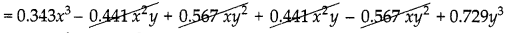= 0.343x3 + 0.729y3

(ii) ($$\frac{1}{2}$$x – $$\frac{1}{2}$$y) ($$\frac{1}{2}$$x2 + $$\frac{1}{2}$$y2)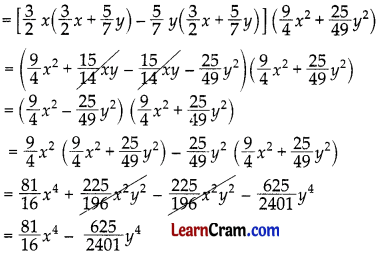Hence verified L.H.S. = R.H.S.

Question 2.
Simplify the following and verify the result for x = 1, y = – 1 and z = 1.
(i) ($$\frac{3}{2}$$x2 – $$\frac{1}{5}$$y) ($$\frac{1}{2}$$x + $$\frac{3}{4}$$xy – $$\frac{1}{3}$$y)
= $$\frac{3}{2}$$x2($$\frac{1}{2}$$x + $$\frac{3}{4}$$xy – $$\frac{1}{3}$$y) – $$\frac{1}{5}$$y($$\frac{1}{2}$$x + $$\frac{3}{4}$$xy – $$\frac{1}{3}$$y)
= $$\frac{3}{4}$$x3 + $$\frac{9}{8}$$x3y – $$\frac{1}{2}$$x2y – $$\frac{1}{10}$$xy – $$\frac{3}{20}$$xy2 + $$\frac{1}{15}$$y2

Verification:
L.H.S = ($$\frac{3}{2}$$x2 – $$\frac{1}{5}$$y) ($$\frac{1}{2}$$x + $$\frac{3}{2}$$xy – $$\frac{1}{3}$$y)
Put x = 1 and y = -1Hence verified L.H.S = R.H.S

(ii) (x2 + y2 + z2 – xy – yz – zx) (x + y + z)
= x2(x + y + z) + y2(x + y + z) + z2 (x + y + z) – xy(x + y + z) – yz (x + y + z) – zx(x + y + z)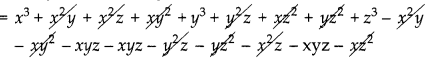= x3 + y3 + z3 – 3xyz

Verification:
L.H.S = (x2 + y2 + z2 – xy – yz – zx) (x + y + z)
= [(1)2 + (-1)2 + (1)2 – (1) (- 1) – (-1) (1) – (1) (1)] [(1) + (-1) + (1)]
= [1 + 1 + 1 + 1 + 1 – 1] [1 – 1 + 1]
= 4 × 1 = 4

R.H.S = x3 + y3 + z3 – 3 xyz
Put x = 1, y = -1, z = 1
= (1)3 + (- 1)3 + (1)3 – 3(1) (- 1) (1)
= 1 – 1 + 1 + 3 = 4
Hence verified L.H.S. = R.H.S.

Question 3.
Simplify the following and verify the result also.
(i) (m3 + n3) (2m – 3n); m = -2, n = 0
(m3 + n3) (2m – 3n)
= m3 (2m – 3n) + n3 (2m – 3n)
= m3 × 2m – m3 × 3n + n3 × 2m – n3 × 3n
= 2m3 – 3m3n + 2mn3 – 3n3

Verification:
L.H.S. = (m3 + n3) (2m – 3n)
Putting m = – 2, n = 0
= [(- 2)3 + (0)3] [2(-2) – 3 × (0)]
= -8 × -4
= 32
R.H.S. = 2m4 – 3m3n + 2mn3 – 3n3
Putting m = -2,n = 0
= 2(- 2)4 – 3 (- 2)3 (0) + 2(- 2) (0)3 – 3 (0)3
= 2 × 16 – 0 + 0 – 0
= 32
Hence verified L.H.S. = R.H.S.

(ii) (x2y – xy2) (2x + 1); x = 1, y = 2
(x2y – xy2) (2x + 1)
= x2y (2x + 1) – xy2(2x + 1)
= x2y × 2x + x2y × 1 – xy2 × 2x – xy2 × 1
= 2x3y + x2y- 2x2 y2 – xy2

Verification:
L.H.S = (x2y – xy2) (2x +1)
Putting x = 1, y = 2
= [(1)2 (2) – (1) (2)2] [2(1) + 1]
= (2 – 4) (2 + 1)
= -2 × 3 = -6

R.H.S = 2x3y + x2y – 2x2y2 – xy2
= 2(1 )3 (2) + (1)2 (2) – 2(1)2 (2)2 – (1) (2)2
= 2× 1 × 2 + 1 × 2 – 2 × 1 × 4 – 1 × 4
= 4 + 2 – 8 – 4
= 6 – 12
= -6
Hence verified L.H.S. – R.H.S.

(iii) (9b2c – 4c2) (2bc2 + 5c); b = 0, c = 2
(9b2c – 4c2) (2bc2 + 5c)
= 9b2c (2bc2 + 5c) – 4c2 (2bc2 + 5c)
= 9b2c x 2bc2 + 9b2c x 5c – 4c2 x 2bc2 – 4c2 x 5c
= 18b3c3 + 45b2c2 – 8bc4 – 20c3

Verification:
L.H.S. = (9b2c – 4c2) (2bc2 + 5c)
Putting b = 0 and c = 2
= [9(0)2 (2) – 4(2)2] [2 × (0) (2)2 + 5(2)]
= [0 – 16] [0 + 10]
= -16 × 10
= – 160

R.H.S. = 18b3c3 + 45b2 – 8bc4 – 20c3
Putting b = 0,c = 2
= 18(0)3 (2)3 + 45(0)2 (2)2 – 8(0) (2)4 – 20(2)3
= 0 + 0 – 0 – 20 × 8
= -160
Hence verified L.H.S. = R.H.S.Question 4.
Find the common factors of the following terms:
(i) 3x3y2,15 xy3, 60x2y2
3x3y2,15xy3, 60x2y2
Factors of 3x3y2 = 3 × x × x × x × y × y
Factors of 15xy3 = 3 × 5 × x × y × y × y
Factors of 60x2y2 = 2 × 2 × 3 × 5 × x × x × y × y
Common factors = 3 × x × y × y = 3xy2

(ii) 4a3b, 12a2b4,16a4b2
4a3b, 12a2b4,16a4b2
Factors of 4 a3b = 2 × 2 × a × a × a × b
Factors of 12 a2b4 = 2 × 2 × 3 × a × a × b × b × b × b
Factors of 16fl4fr2 = 2 × 2 × 2 × 2 × a × a × a × a × b × b
Common factors are 2 × 2 × a × a × b = 4 a2b

(iii) 8x2(x – y), 12x(x – y), 24x3y2 (x – y)
8x2(x – y), 12x(x – y), 24x3y2 (x – y)
Factors of 8x2(x – y) = 2 × 2 × 2 × x × x × x – y)
Factors of 12x(x – y) =2 × 2 × 3 × x × (x – y)
Factors of 24x3y2 (x – y) = 2 × 2 × 2 × 3 × x × x × x × y × y × (x – y)
Common factors are 2 × 2 × x × (x – y) = 4x(x – y)

(iv) 21x3y7z2, 35x2 y6z3, 42x5y3z4
21x3y7z2, 35x2 y6z3, 42x5y3z4
Factors of 21x3y7z2 = 3 × 7 × x × x × x × y × y × y × y × y × y × y × z × z
Factors of 35x2 y6z3 = 5 × 7 × x × x × y × y × y × y × y × y × z × z × z
Factors of 42x5y3z4 = 2 × 3 × 7 × x × x × x × x × x × y × y × y × z × z × z × z
Common Factors are 7 × x × x × y × y × y × z × z = 7x2y3z2

Question 5.
Find H.C.F. of the terms and factorise the following expressions.
(i) 21x4y5 + 28x5y3 – 35x3y7
21x4y5 + 28x5y3 – 35x3y7
Common factors = 7x3y3
H.C.F. of the terms = 7x3y3
∴ 21x4y5 + 28x5y3 – 35 x3y7 = 7x3y3(3xy3 + 4x2 – 5y4)

(ii) 5p3q – 20pq3 + 3p2q2
5p3q – 20pq3 + 3p2q2
Common factor = pq
∴ H.C.F. of the terms = pq
∴ 5p3q – 20pq3 + 3p2q2 = pq(5p2 + 4x2 – 5y4)

Question 6.
Factorise the following expressions:
(i) (3x – 5y)2 – 15x2y + 25xy2
(3x – 5y)2 – 15x2y + 25xy2
= (3x – 5y)2 – 5xy (3x – 5y)
= (3x – 5y) (3x – 5y – 5xy)

(ii) 9x2 – 16y2 + 18x3y – 12xy3
9x2 – 16y2 + 18x3y – 12xy3
= (3x)2 – (4y)2 + 6xy (3x – 4y)
= (3x – 4y) (3x + 4y) + 6xy (3x – 4y)
= (3x – 4y) (3x + 4y + 6xy)Question 7.
Simplify the following and verify the result for the given values:
(i) (2a – 3b) (4a2 + 6ab + 9b2); a = 1, b = 2
(2a – 3b) (4a2 + 6ab + 9b2) = 2a(4a2 + 6ab + 9b2) – 3b(4a2 + 6ab + 9b2)= 8a3 – 27b3

Verification:
L.H.S. = (2a – 3b) (4a2 + 6ab + 9b2)
Put a = 1,b = 2
= [2(1)-3 (2)] [4 (1)2 + 6(1)(2) + 9 (2)2]
= (2 – 6) (4 + 12 + 36)
= – 4 × 52 = – 208
= 8a3 – 27b3

R.H.S
Put x = 1, b = 2
= 8(1)3 – 27 (2)3
= 8 – 27 × 8
= 8 – 216
= -208
Hence verified L.H.S = R.H.S

(ii) ($$\frac{1}{3}$$p2 – $$\frac{2}{5}$$q2) (p + q – pq); p = -1, q = 1
($$\frac{1}{3}$$p2 – $$\frac{2}{5}$$q2) (p + q – pq)
= $$\frac{1}{3}$$p2(p + q – pq) + $$\frac{2}{5}$$q2(p + q – pq)
= $$\frac{1}{3}$$p3 + $$\frac{1}{3}$$p2q – $$\frac{1}{3}$$p3q + $$\frac{2}{5}$$pq2 + $$\frac{2}{5}$$q3 – $$\frac{2}{5}$$pq3

Verification:
L.H.S. = ($$\frac{1}{3}$$p2 – $$\frac{2}{5}$$q2)
Put p = -1, q = 1
= [$$\frac{1}{3}$$(- 1)2 + $$\frac{2}{5}$$(1)2] [-1 + 1 – (- 1) (1)]
= $$\left(\frac{1}{3}+\frac{2}{5}\right)$$(-1 + 1 + 1)
= $$\left(\frac{5+6}{15}\right)$$(1)
= $$\frac{11}{15}$$

R.H.S.
= $$\frac{1}{3}$$p3 + $$\frac{1}{3}$$p2q – $$\frac{1}{3}$$p3q + $$\frac{2}{5}$$pq2 + $$\frac{2}{5}$$q3 – $$\frac{2}{5}$$pq3

Put p = – 1 and q = 1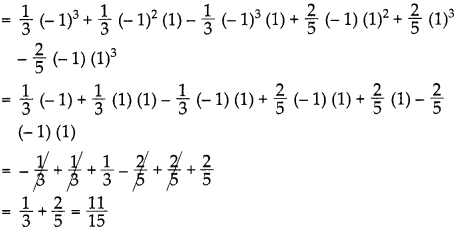Hence verified L.H.S. = R.H.S.Question 8.
Factorise:
(i) pxy + qr xy – pz – qrz